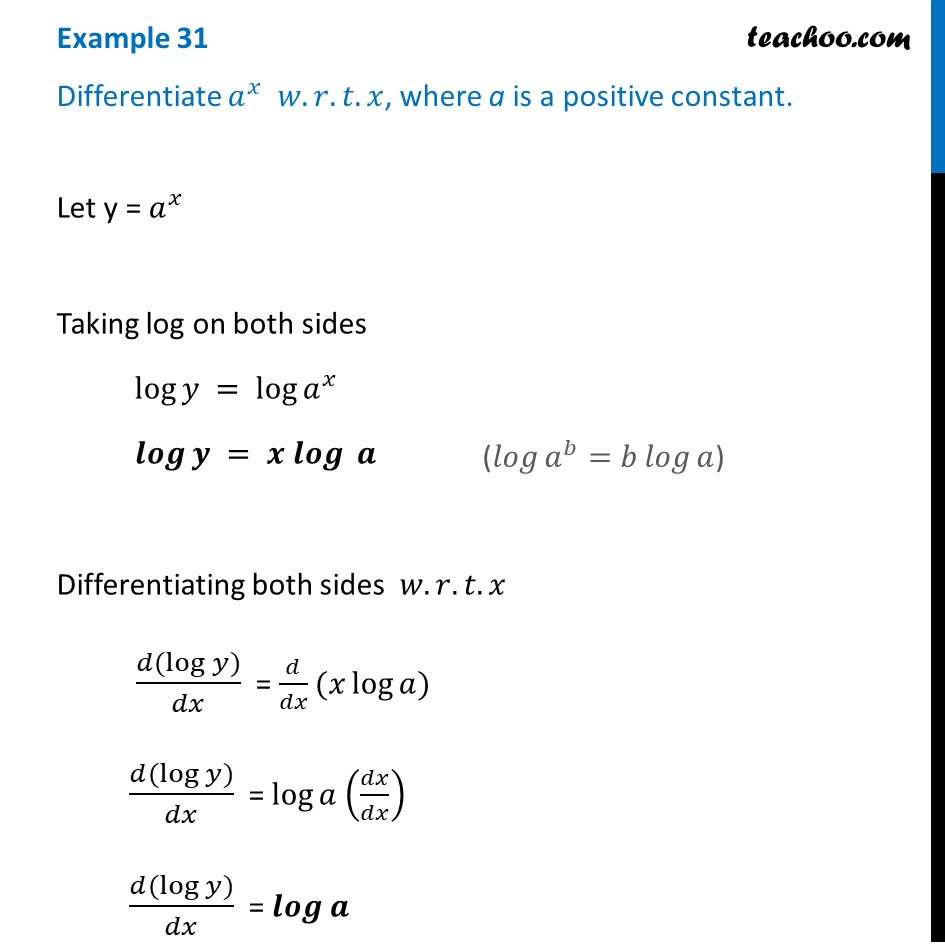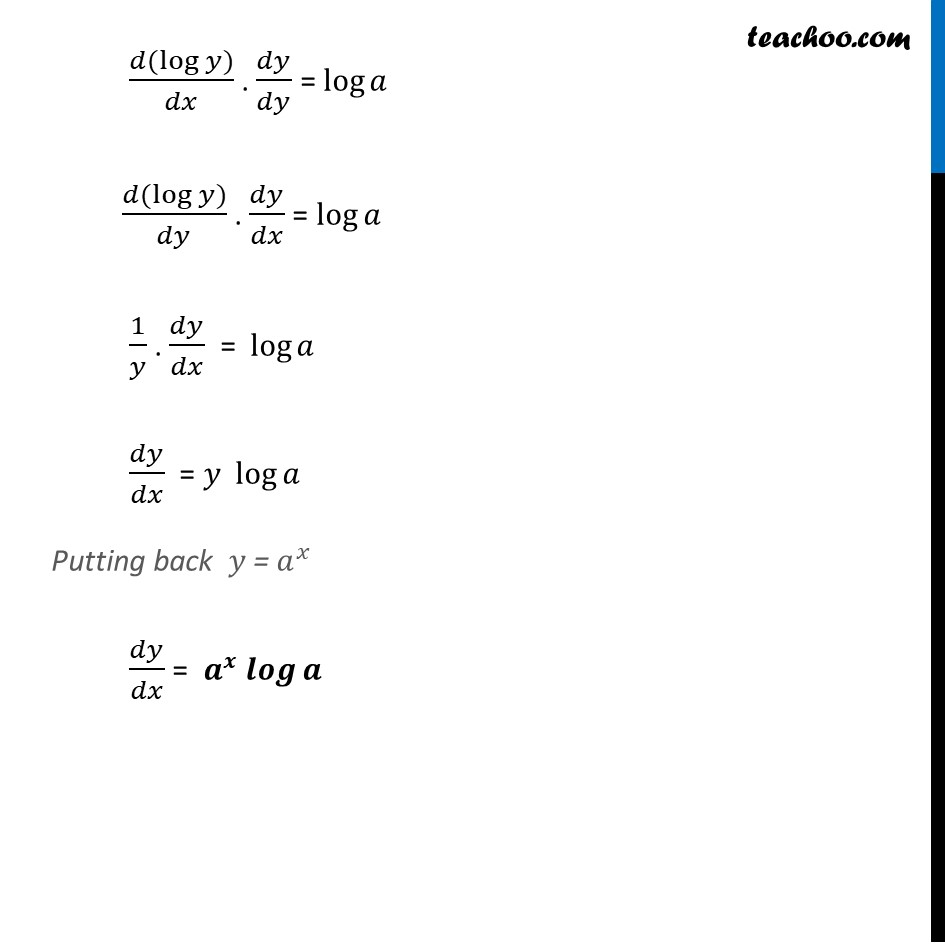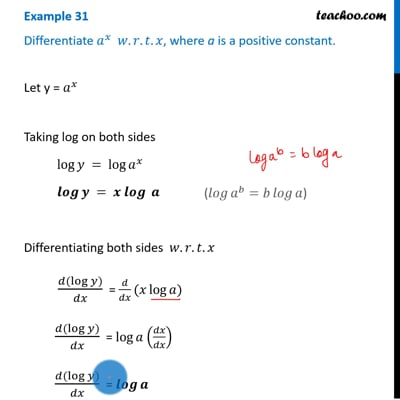Logarithmic Differentiation - Type 1

Chapter 5 Class 12 Continuity and Differentiability
Concept wiseThis video is only available for Teachoo black users

Introducing your new favourite teacher - Teachoo Black, at only ₹83 per month

### Transcript

Example 31 Differentiate 𝑎^𝑥 𝑤.𝑟.𝑡.𝑥, where a is a positive constant.Let y = 𝑎^𝑥 Taking log on both sides log⁡𝑦 = log⁡𝑎^𝑥 𝒍𝒐𝒈⁡𝒚 = 𝒙 𝒍𝒐𝒈⁡ 𝒂 Differentiating both sides 𝑤.𝑟.𝑡.𝑥 (𝑑(log⁡𝑦))/𝑑𝑥 = 𝑑/𝑑𝑥(𝑥 log⁡𝑎) (𝑑(log⁡𝑦))/𝑑𝑥 = log⁡𝑎 (𝑑𝑥/𝑑𝑥) (𝑑(log⁡𝑦))/𝑑𝑥 = 𝒍𝒐𝒈⁡𝒂 (𝑙𝑜𝑔⁡〖𝑎^𝑏=𝑏 𝑙𝑜𝑔⁡𝑎 〗) (𝑑(log⁡𝑦))/𝑑𝑥 . 𝑑𝑦/𝑑𝑦 = log⁡𝑎 (𝑑(log⁡𝑦))/𝑑𝑦 . 𝑑𝑦/𝑑𝑥 = log⁡𝑎 1/𝑦 . 𝑑𝑦/𝑑𝑥 = log⁡𝑎 𝑑𝑦/𝑑𝑥 = 𝑦 log⁡𝑎 Putting back 𝑦 = 𝑎^𝑥 𝑑𝑦/𝑑𝑥 = 𝒂^𝒙 𝒍𝒐𝒈⁡𝒂